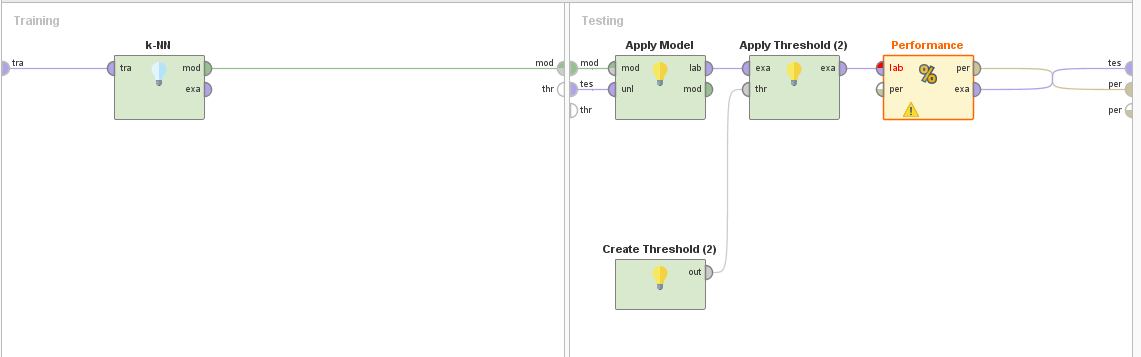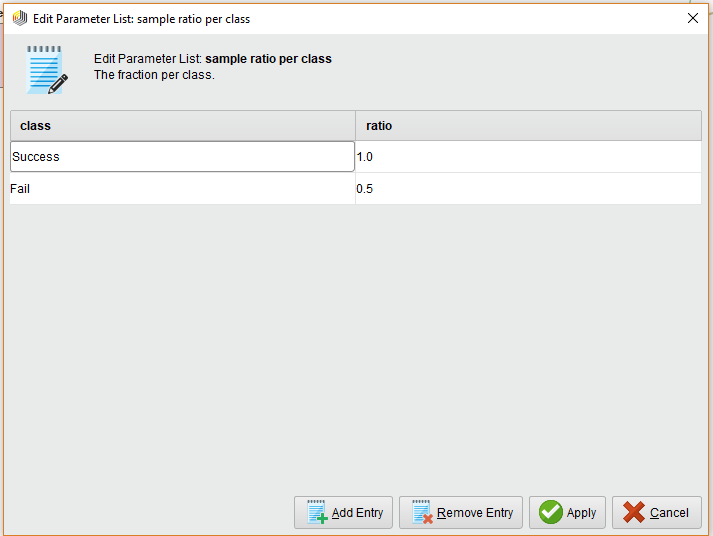# Optimize Auto Model towards Sensitivity

Member Posts: 2Newbie
edited June 2019 in Help
Hi Experts,

Some context on my problem: I have an unbalanced dataset with 3k observations from which about 5% are successful companies and 95% unsuccessful ones. The underlying definitions of successful/failure are not relevant here as the dataset contains only labels 0 (failure) or 1 (successful). For every company, I have about 150 features which were identified at point in time t1. The label successful/unsuccessful was identified at point in time t2 because at point t1 it's unclear whether the company will become successful or not.

Goal: Based on the information we have at point t1, I want to predict whether the company will become a success or failure at point t2. The model should serve as a pre-selection tool for venture capital investors to figure out on which companies to focus their attention, i.e., which have the highest likelihood of success. In venture capital, only very small number of portfolio companies account for the majority of the fund's return. The majority of companies are failures and don't return anything. The return distribution is similar to a pareto distribution where 20% of companies account for 80% of returns. Consequently, the investor cannot afford to miss out on any of the success cases. This means that while it's okay to wrongly classify failures as success, it's not okay to wrongly classify a success as a failure, i.e., I need to optimise the model towards sensitivity (avoid false negatives).

Problem: After running the Auto Model, I have 2 questions: 1) With the default setting only Naive Bayes leads to a sensitivity different to 0, i.e., 87.5%. How can I optimize all models towards sensitivity? 2) How can I limit the number of success predictions? Once I want to optimize the model towards sensitivity (avoid false negatives), the model could easily predict every company as success and end up with 100% sensitivity. Is it possible to limit the number of success predictions to a specific threshold, e.g., 20% of the sample size?

Tagged:

• Moderator, RapidMiner Certified Analyst, Member Posts: 1,195Unicorn
HI @VCResearcher_0,

By default, Auto-Model is optimizing a model based on the accuracy....
After opening each process (for example the process associated to a Decision Tree model) generated by Auto-Model, you have to :
- Go inside the Optimize Parameters operator -> Cross Validation operator
- Replace the Performance operator by Performance (Binominal Classification) operator
- Set sensitivity in the main criterion parameter of this operator.
This time, RapidMiner will optimize the parameter(s) of your model to maximize the sensitivity.

I hope it helps,

Regards,

Lionel

• Moderator, RapidMiner Certified Analyst, Member Posts: 1,195Unicorn
Hi again @VCResearcher_0,

By defaut, for a binary classification problem, RapidMiner apply a threshold of 0,5 on the confidences
to determine the predicted class...
To modify (increase) this threshold, you can use the association Create Threshold / Apply Threshold operators like this :I propose you increase this threshold, for example threshold = 0,7. In this case, you will have :
If confidence(target = Success) > 0,7, then predicted class = Success
else predicted class = Fail

There is no "automatic way" in RapidMiner to obtain / calculate the threshold corresponding to a final sensitivity of  20%.
Logically, the more you increase the threshold (0,7 - 0,8 - 0,9 - 0,95 etc.) the more the sensitivity decrease..
It's up to you to adjust by dichotomy the threshold to obtain a sensitivity of 20%.

I hope it helps,

Regards,

Lionel

• Member Posts: 2Newbie
Thank you, Lionel!

Re your first answer, it only changed the confusion matrix results for the Gradient Boosted Trees (i.e., when opening the generated model in "Design" >> "Optimize Parameters" >> "Cross Validation" >> "Inner Performance (Bin. Class.)" and changing the main criterion to "sensitivity", it jumped from 0% to 18.75%). Unfortunately, results did not change at all for Decision Trees and Random Forest (and I actually could not find the respective operator for Deep Learning, Log Reg, Gen Reg and Naive Bayes). This feels a bit weird though. What do you think?

Re your second answer, I don't want to limit the sensitivity (it should be actually maximized as much as possible) but limit the number of positive predictions, i.e., only predict 20% (or for a dataset of 200 observations only predict 40 observations) as success but with the highest sensitivity possible. Is there a way to limit the number of success predictions but still maximum sensitivity?

The ultimate goal is to find a model which has the highest sensitivity and can be limited in the number of positive (success) predictions. Any ideas?
• Moderator, RapidMiner Certified Analyst, Member Posts: 1,195Unicorn
Hi @VCResearcher_0,

1. It's the expected behaviour of Auto Model :
By default, Auto Model don't perform parameters optimization for the models you mentionned. To optimize these models
you have to open the generated process and manually add an Optimize Parameters operator (inspire you to the model of Decision Tree for example).

2. To increase the sensitivity, you can sample your dataset with the Sample operator.By this way, you increase the ratio success / fail in the training set used to train your model(s) and then you increase the sensitivity.
It's up to you to adjust by dichotomy these 2 ratios to maximize the sensitivity and simultaneously obtain a success prediction rate of 20%.

I hope it helps,

Regards,

Lionel

NB : Process with Sample operator to inspire you :
```<?xml version="1.0" encoding="UTF-8"?><process version="9.1.000">
<context>
<input/>
<output/>
<macros/>
</context>
<operator activated="true" class="process" compatibility="9.1.000" expanded="true" name="Process">
<parameter key="logverbosity" value="init"/>
<parameter key="random_seed" value="2001"/>
<parameter key="send_mail" value="never"/>
<parameter key="process_duration_for_mail" value="30"/>
<parameter key="encoding" value="SYSTEM"/>
<process expanded="true">
<operator activated="true" breakpoints="after" class="retrieve" compatibility="9.1.000" expanded="true" height="68" name="Retrieve Titanic" width="90" x="45" y="85">
<parameter key="repository_entry" value="//Samples/data/Titanic"/>
</operator>
<operator activated="true" class="set_role" compatibility="9.1.000" expanded="true" height="82" name="Set Role" width="90" x="179" y="85">
<parameter key="attribute_name" value="Survived"/>
<parameter key="target_role" value="label"/>
<parameter key="Name" value="id"/>
</list>
</operator>
<operator activated="true" breakpoints="after" class="sample" compatibility="9.1.000" expanded="true" height="82" name="Sample" width="90" x="380" y="85">
<parameter key="sample" value="relative"/>
<parameter key="balance_data" value="true"/>
<parameter key="sample_size" value="100"/>
<parameter key="sample_ratio" value="0.1"/>
<parameter key="sample_probability" value="0.1"/>
<list key="sample_size_per_class">
<parameter key="yes" value="500"/>
<parameter key="no" value="500"/>
</list>
<list key="sample_ratio_per_class">
<parameter key="Yes" value="1.0"/>
<parameter key="No" value="0.5"/>
</list>
<list key="sample_probability_per_class"/>
<parameter key="use_local_random_seed" value="false"/>
<parameter key="local_random_seed" value="1992"/>
</operator>
<operator activated="true" class="concurrency:cross_validation" compatibility="9.1.000" expanded="true" height="145" name="Cross Validation" width="90" x="581" y="85">
<parameter key="split_on_batch_attribute" value="false"/>
<parameter key="leave_one_out" value="false"/>
<parameter key="number_of_folds" value="10"/>
<parameter key="sampling_type" value="automatic"/>
<parameter key="use_local_random_seed" value="false"/>
<parameter key="local_random_seed" value="1992"/>
<parameter key="enable_parallel_execution" value="true"/>
<process expanded="true">
<operator activated="true" class="k_nn" compatibility="9.1.000" expanded="true" height="82" name="k-NN" width="90" x="179" y="34">
<parameter key="k" value="5"/>
<parameter key="weighted_vote" value="true"/>
<parameter key="measure_types" value="MixedMeasures"/>
<parameter key="mixed_measure" value="MixedEuclideanDistance"/>
<parameter key="nominal_measure" value="NominalDistance"/>
<parameter key="numerical_measure" value="EuclideanDistance"/>
<parameter key="divergence" value="GeneralizedIDivergence"/>
<parameter key="kernel_gamma" value="1.0"/>
<parameter key="kernel_sigma1" value="1.0"/>
<parameter key="kernel_sigma2" value="0.0"/>
<parameter key="kernel_sigma3" value="2.0"/>
<parameter key="kernel_degree" value="3.0"/>
<parameter key="kernel_shift" value="1.0"/>
<parameter key="kernel_a" value="1.0"/>
<parameter key="kernel_b" value="0.0"/>
</operator>
<connect from_port="training set" to_op="k-NN" to_port="training set"/>
<connect from_op="k-NN" from_port="model" to_port="model"/>
<portSpacing port="source_training set" spacing="0"/>
<portSpacing port="sink_model" spacing="0"/>
<portSpacing port="sink_through 1" spacing="0"/>
</process>
<process expanded="true">
<operator activated="true" class="apply_model" compatibility="9.1.000" expanded="true" height="82" name="Apply Model" width="90" x="45" y="34">
<list key="application_parameters"/>
<parameter key="create_view" value="false"/>
</operator>
<operator activated="true" class="performance_binominal_classification" compatibility="9.1.000" expanded="true" height="82" name="Performance" width="90" x="179" y="34">
<parameter key="main_criterion" value="first"/>
<parameter key="accuracy" value="true"/>
<parameter key="classification_error" value="false"/>
<parameter key="kappa" value="false"/>
<parameter key="AUC (optimistic)" value="false"/>
<parameter key="AUC" value="false"/>
<parameter key="AUC (pessimistic)" value="false"/>
<parameter key="precision" value="false"/>
<parameter key="recall" value="false"/>
<parameter key="lift" value="false"/>
<parameter key="fallout" value="false"/>
<parameter key="f_measure" value="false"/>
<parameter key="false_positive" value="false"/>
<parameter key="false_negative" value="false"/>
<parameter key="true_positive" value="false"/>
<parameter key="true_negative" value="false"/>
<parameter key="sensitivity" value="true"/>
<parameter key="specificity" value="false"/>
<parameter key="youden" value="false"/>
<parameter key="positive_predictive_value" value="false"/>
<parameter key="negative_predictive_value" value="false"/>
<parameter key="psep" value="false"/>
<parameter key="skip_undefined_labels" value="true"/>
<parameter key="use_example_weights" value="true"/>
</operator>
<connect from_port="model" to_op="Apply Model" to_port="model"/>
<connect from_port="test set" to_op="Apply Model" to_port="unlabelled data"/>
<connect from_op="Apply Model" from_port="labelled data" to_op="Performance" to_port="labelled data"/>
<connect from_op="Performance" from_port="performance" to_port="performance 1"/>
<portSpacing port="source_model" spacing="0"/>
<portSpacing port="source_test set" spacing="0"/>
<portSpacing port="source_through 1" spacing="0"/>
<portSpacing port="sink_test set results" spacing="0"/>
<portSpacing port="sink_performance 1" spacing="0"/>
<portSpacing port="sink_performance 2" spacing="0"/>
</process>
</operator>
<connect from_op="Retrieve Titanic" from_port="output" to_op="Set Role" to_port="example set input"/>
<connect from_op="Set Role" from_port="example set output" to_op="Sample" to_port="example set input"/>
<connect from_op="Sample" from_port="example set output" to_op="Cross Validation" to_port="example set"/>
<connect from_op="Cross Validation" from_port="model" to_port="result 1"/>
<connect from_op="Cross Validation" from_port="performance 1" to_port="result 2"/>
<portSpacing port="source_input 1" spacing="0"/>
<portSpacing port="sink_result 1" spacing="0"/>
<portSpacing port="sink_result 2" spacing="0"/>
<portSpacing port="sink_result 3" spacing="0"/>
</process>
</operator>
</process>
```